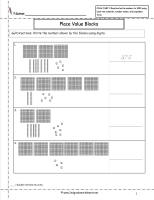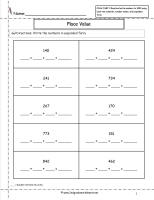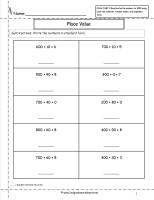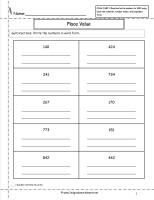﻿ CCSS 2.NBT.3 Worksheets.  Place Value Worksheets-Read and Write Numbers

# Common Core State Standard 2.NBT.3

## Number & Operations in Base Ten

### Understand Place Value.

3. Read and write numbers to 1000 using base-ten numerals, number names, and expanded form.

###Place Value Blocks Write the Number23

Common Core State Standards: 2.NBT.3
Numbers & Operations in Base Ten
Understand Place Value.

Read and write numbers to 1000 using base-ten numerals, number names, and expanded form.

Students write the number shown by the blocks.

###Place Value Worksheets234 5

Common Core State Standards: 2.NBT.3
Numbers & Operations in Base Ten
Understand Place Value.

Read and write numbers to 1000 using base-ten numerals, number names, and expanded form.

Students write the numbers in expanded notation.

###Place Value  Worksheets 2345

Common Core State Standards: 2.NBT.3
Numbers & Operations in Base Ten
Understand Place Value.

Read and write numbers to 1000 using base-ten numerals, number names, and expanded form.

Students change numbers from expanded notation to standard notation.

###Place Value Worksheets2345

Common Core State Standards: 2.NBT.3
Numbers & Operations in Base Ten
Understand Place Value.

Read and write numbers to 1000 using base-ten numerals, number names, and expanded form.

Students write the numbers from word form to standard form.

###Place Value Worksheets 2345

Common Core State Standards: 2.NBT.3
Numbers & Operations in Base Ten
Understand Place Value.

Read and write numbers to 1000 using base-ten numerals, number names, and expanded form.

Students write the numbers in word form.